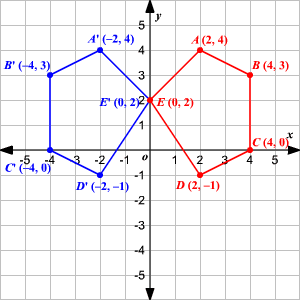# Transformation of Graphs Using Matrices - Reflection

A reflection is a transformation representing a flip of a figure. Figures may be reflected in a point, a line, or a plane. When reflecting a figure in a line or in a point, the image is congruent to the preimage.

A reflection maps every point of a figure to an image across a line of symmetry using a reflection matrix.

Use the following rule to find the reflected image across a line of symmetry using a reflection matrix.

$\begin{array}{|cccc|}\hline \hfill \text{For}\text{\hspace{0.17em}}\text{\hspace{0.17em}}\text{a}\text{\hspace{0.17em}}\text{\hspace{0.17em}}\text{reflection}\text{\hspace{0.17em}}\text{\hspace{0.17em}}\text{over}\text{\hspace{0.17em}}\text{\hspace{0.17em}}\text{the}:& \hfill x-\text{axis}& \hfill y-\text{axis}& \hfill \text{line}\text{\hspace{0.17em}}\text{\hspace{0.17em}}y=x\\ \hfill \begin{array}{l}\hfill \text{Multiply}\text{\hspace{0.17em}}\text{\hspace{0.17em}}\text{the}\text{\hspace{0.17em}}\text{\hspace{0.17em}}\text{vertex}\\ \hfill \text{on}\text{\hspace{0.17em}}\text{\hspace{0.17em}}\text{the}\text{\hspace{0.17em}}\text{\hspace{0.17em}}\text{left}\text{\hspace{0.17em}}\text{\hspace{0.17em}}\text{by}\end{array}& \hfill \left[\begin{array}{cc}\hfill 1& \hfill 0\\ \hfill 0& \hfill -1\end{array}\right]& \hfill \left[\begin{array}{cc}\hfill -1& \hfill 0\\ \hfill 0& \hfill 1\end{array}\right]& \hfill \left[\begin{array}{cc}\hfill 0& \hfill 1\\ \hfill 1& \hfill 0\end{array}\right]\\ \hline\end{array}$

Example:

Find the coordinates of the vertices of the image of pentagon $ABCDE$ with $A\left(2,4\right),B\left(4,3\right),C\left(4,0\right),D\left(2,-1\right),\text{\hspace{0.17em}}\text{\hspace{0.17em}}\text{and}\text{\hspace{0.17em}}\text{\hspace{0.17em}}E\left(0,2\right)$ after a reflection across the $y$ -axis.

Write the ordered pairs as a vertex matrix.

$\left[\begin{array}{ccccc}\hfill 2& \hfill 4& \hfill 4& \hfill 2& \hfill 0\\ \hfill 4& \hfill 3& \hfill 0& \hfill -1& \hfill 2\end{array}\right]$

To reflect the pentagon $ABCDE$ across the $y$ -axis, multiply the vertex matrix by the reflection matrix $\left[\begin{array}{cc}\hfill -1& \hfill 0\\ \hfill 0& \hfill 1\end{array}\right]$ .

$\left[\begin{array}{cc}\hfill -1& \hfill 0\\ \hfill 0& \hfill 1\end{array}\right]\cdot \left[\begin{array}{ccccc}\hfill 2& \hfill 4& \hfill 4& \hfill 2& \hfill 0\\ \hfill 4& \hfill 3& \hfill 0& \hfill -1& \hfill 2\end{array}\right]=\left[\begin{array}{ccccc}\hfill -2& \hfill -4& \hfill -4& \hfill -2& \hfill 0\\ \hfill 4& \hfill 3& \hfill 0& \hfill -1& \hfill 2\end{array}\right]$

Therefore, the coordinates of the vertices of the image of pentagon $ABCDE$ are ${A}^{\text{'}}\left(-2,4\right),{B}^{\text{'}}\left(-4,3\right),{C}^{\text{'}}\left(-4,0\right),{D}^{\text{'}}\left(-2,-1\right),\text{\hspace{0.17em}}\text{\hspace{0.17em}}\text{and}\text{\hspace{0.17em}}\text{\hspace{0.17em}}{E}^{\text{'}}\left(0,2\right)$ .Notice that, both figures have the same size and shape.